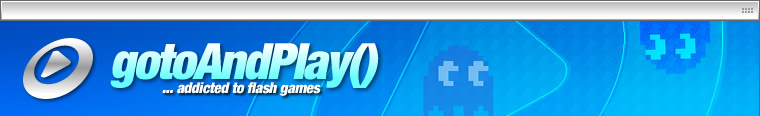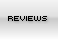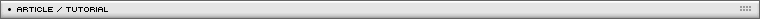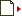Moving sprites
[ September 15, 2003 ] by Mad Sci
How to move clips around the stage using the keyboard.

With this tutorial I will try to explain different types of movement using the keyboard events. We will start with simple scripts and finish with more advanced scripting technique. I will assume that you already have good ammout of knowlege about Flash and Flash 5.0 Action Scripting. Lets start with something simple.

Set your stage to be 300 x 300 pixels.

Create a MC on the stage. Now right mouse click to bring up the Action Script window. What we want is to move this clip when the user presses the RIGHT, LEFT, UP or DOWN key. Here is what we do:

```onClipEvent(enterFrame)
{
if (Key.isDown (Key.RIGHT)) this._x+=5;
if (Key.isDown (Key.LEFT)) this._x-=5;
if (Key.isDown (Key.UP)) this._y-=5;
if (Key.isDown (Key.DOWN)) this._y+=5;
}```

Now keep in mind that the upper left hand corner has coordinates 0,0 and the lower right hand corner is with 300,300. Note that when you press the LEFT and UP buttons at the same time the movement of this clip is kinda jearky. To overcome this problem we will use the ELSE statement. What this statement will do is every time the user presses a key the rest of the key events will be ignored, so one key at a time.

```onClipEvent(enterFrame)
{
if (Key.isDown (Key.RIGHT)) this._x+=5;
else if (Key.isDown (Key.LEFT)) this._x-=5;
else if (Key.isDown (Key.UP)) this._y-=5;
else if (Key.isDown (Key.DOWN)) this._y+=5;
}```

Note that the MC will move around the stage with a step of 5 pixels / frame. If you run your movie at speed of 30 fpm this movement will be more smooth and fast. At any given time you may want to change the step, we can do this by puting additional variable STEP.

```onClipEvent(load) step=5;
// I use onClipEvent load to init some variables. This line will be executed when the clip loads

onClipEvent(enterFrame)
{
if (Key.isDown (Key.RIGHT)) this._x+=step;
else if (Key.isDown (Key.LEFT)) this._x-=step;
else if (Key.isDown (Key.UP)) this._y-=step;
else if (Key.isDown (Key.DOWN)) this._y+=step;
}```

So far so good. Still one problem exists. What if you move the MC off the stage? As we said before the upper left hand corner of the stage will have coordinates 0,0 and the lower righthand corner will be 300,300 (if you set your movie 300 x 300). So the TOP border will have Y=0, the LOWER border Y=300, LEFT border X=0, RIGHT border Y=300. If at any given moment the movie clip has X and Y values smaller than thouse it can move.

```onClipEvent(load) step=5;

onClipEvent(enterFrame)
{
if (Key.isDown (Key.RIGHT) && this._x < 300) this._x+=step;
else if (Key.isDown (Key.LEFT) && this._x > 0) this._x-=step;
else if (Key.isDown (Key.UP) && this._y > 0) this._y-=step;
else if (Key.isDown (Key.DOWN) && this._y < 300) this._y+=step;
}```

If you have any problem understanding the coordinate system above you can use this code.

```onClipEvent(load)
{
step=5;
movieWidth=300;
movieHeight=300;
}

onClipEvent(enterFrame)
{
if (Key.isDown (Key.RIGHT) && this._x < movieWidth) this._x+=step;
else if (Key.isDown (Key.LEFT) && this._x > 0) this._x-=step;
else if (Key.isDown (Key.UP) && this._y > 0) this._y-=step;
else if (Key.isDown (Key.DOWN) && this._y < movieHeight) this._y+=step;
}```

The above code will be useless if we dont put some animations. There are 2 ways of animating a movie.

1) Use keyframes in the MC which you move.
2) Use .attachMovie

I personaly use attachMovie. What this command will do is to pull MC from your library and attach them to the MC which you are moving. I think that this method is more faster and does not glog the memory too much. Here is how is done.

On the stage create a square and conver to MC. Give it name sqr. Open your library and create a folder named Hero. This folder will contan all MC which you will be using for the hero animation. Move the sqr MC in this folder. Now Right Mouse click over the MC sqr in the library and sellect LINKAGE. Select Export this symbol radio button and give it a name sqr. Now delete the MC sqr on the stage. All you need is to have it in the library. Here is how we are going to attach this MC to our MC containing the above moving script.

.attachMovie(idName, newName, depth)

Where:
- idName: he name you used for the MC when you selected LINKAGE (see above);
- newName: what will be the name of the attached movie clip;
- depth: what will be the depth of the MC (depth is like layer in Adobe Photoshop).

```onClipEvent(load)
{
step=5;
movieWidth=300;
movieHeight=300;
this.stop();
}

onClipEvent(enterFrame)
{
if (Key.isDown (Key.RIGHT) && this._x < movieWidth)
{
this.attachMovie("sqr", "sqr1", 10 );
this._x+=step;
}
else if (Key.isDown (Key.LEFT) && this._x > 0) this._x-=step;
else if (Key.isDown (Key.UP) && this._y > 0) this._y-=step;
else if (Key.isDown (Key.DOWN) && this._y < movieHeight) this._y+=step;
}```

Now when you press the RIGHT key button the clip will change to sqr. Note the stop() action which I used. Because this MC is empty there is no need to play it. This will save us memory. From the other side the MC wich you attach to this MC can have animations. It is a good idea if you give instance name of your main movie. I use the name container; empty MC with script only. If you did animations for LEFT, RIGHT, UP, DOWN and you want to dislplay them when the appropriate button is down use the same trick as above but keep this in mind. The depth should be the same for all animations. In this case I used depth of 10, so all animation which will be attached to this MC will have one and the same depth or each movie will unload the previous. :-)

this.attachMovie("run_left", "run", 10 )
this.attachMovie("run_right", "run", 10 )
this.attachMovie("run_up", "run", 10 )
this.attachMovie("run_down", "run", 10 )

PLATFORM GAMES: JUMPING

In platform games like (Mario, LodeRunner, Donkey Kong, etc.) the movements could be summarized as: LEFT, RIGHT, JUMPING, CLIMBING, FALLING, CROUCHING. The first 2 LEFT and RIGHT were already discussed above. Now lets see how to do JUMPING. In platform games the most important question is WHERE IS THE GROUND? Now imagine yourself jumping. First you are on the ground, then there is rapid push upwards. At that moment your speed is bigger than the Earths gravity and you will move fast. Gradually your upward speed will decline due to the oposing gravity force. At that very moment just for few milisec. will look as you are hanging in the air, this is when your speed becomes 0, then you will start going back to Earth and your speed gradually will incrase. How do we simulate that in Flash:

1. Create a MC on the stage with W:190 and H:20. Give it instance name ground. This MC will play the ground in our case.
2. Create a circle MC W:15 H:15 and put it right on top of the ground. This will be our ball MC. This ball will jump when we press SPACE.

The initial speed of the ball will have a negative value when it hits 0 it will become with positive value wich gradually increses. We also want to check when the SPACE is down, but only once. If the user keeps the SPACE down it will be ignored. You cannot jump while in air.
Get the ball MC AS window and paste this code:

```onClipEvent(load)
{
vel_y = 0;
jumping = false;
}

onClipEvent(enterFrame)
{
if (Key.isDown(Key.SPACE) && !jumping)
{
vel_y = 10;
jumping = true;
}
if (jumping)
{
vel_y -= 1;
if (vel_y <= -10) vel_y =- 10;
this._y -= vel_y;
}
if (_root.ground.hitTest(this._x, this._y+15, true))
{
vel_y = 0;
jumping = false;
}
}```

Now lets look in the above code: when the clip loads 2 variables will be initialized.

1) vel_y = 0 means velocity of the jump = 0 or we are not moving;
2) jumping = false is a boolean variable which says that we are currantly not jumping.

The next thing which the script will do is to look if the SPACE key is pressed and if we are not jumping. If we are currantly jumping, or the variable jumping = true this line will be ignored, so we cannot jump while in air. If we are on the ground the line will be executed and again 2 variables are initialized.

1) vel_y = 10 is how high the clip will jump. Play with that variable give it different values to see the effect;
2) jumping = true indicates that the jumping was initiated.

So what will happen if we want to jump as indicated by jumping = true. The variable vel_y will start to decline, but since the _y -= vel_y the MC will actually move up. In this line: vel_y -= 1; the 1 is actually the acceleration of the jump. Now if the MC does not hit the ground it will continue to fall with increasing speed. We want to limit this by using: if (vel_y <= -10){ vel_y=-10;} This will indicate that the MC is falling with maximum speed. The last line actually detects if we hit the ground. This line is actually looking 15 pixels bellow the center if there is a collision with the ground. If thats the case, then we restore the variables vel_y = 0 (we stopped the move) and jumping = false (so we can jump again).

PLATFORM GAMES: FALLING OFF A PLATFORM

As we said above knowing were the ground is is the most important thing in the platform games. So how a MC would know when it should fall off a platform. The most common approach is to look right under the MC to see if there is a collision with the ground MC. Lets see how to simulate this.

1) Create 2 MC: W:120 H:20. Put them in a such a way that when you walk of the top platform you will land on the second one. Now give them instance names g1 for the top platform and g2 for the one bellow.
2) Create a ball MC W:15 H:15. and put it on top of the platform g1.

```onClipEvent(load)
{
grav = 0;
vel = 1;
}

onClipEvent(enterFrame)
{
fall = true;
if (_root.g1.hitTest(this._x, this._y+15, true))
{
fall = false;
vel = 1;
grav = 0;
}
if (_root.g2.hitTest(this._x, this._y+15, true))
{
fall = false;
vel = 1;
grav = 0;
}
if (Key.isDown(Key.RIGHT)) this._x+=5;
if (fall)
{
grav += vel;
vel += 1;
if (grav >= 14) grav = 14;
this._y += grav;
}
}```

Now lets look in this script. When loaded the script will init 2 variables grav and vel. The script will assume that we are actually falling fall = true. Then it will check if there is collision 15 pixels bellow the center of the ball MC. If that is true than it will make the variable fall = false; no velocity or gravity. In case that we are falling or walking off the platform: if (fall){ the MC will start falling with increasing speed: grav += vel; vel += 1; again vel += 1 where 1 is actually the acceleration of the fall.

In overhead view LEFT and RIGHT mouse keys will rotate the hero left and right, when pressign the UP key the hero will move in that direction.
Create an Arrow movie clip wich points at 3 o'clock. Get the actions and paste the following code.

```onClipEvent(load)
{
speed = 5;
angle = 30;
}

onClipEvent(enterFrame)
{
if (Key.isDown(Key.UP))
{
xx = math.cos(_rotation*Math.PI/180)*speed;
yy = math.sin(_rotation*Math.PI/180)*speed;
this._x += xx;
this._y += yy;
}
if (Key.isDown(Key.LEFT))
{
this._rotation -= angle;
key_down = 1;
}
else if (Key.isDown(Key.RIGHT))
{
key_down = 1;
this._rotation += angle;
}
}```

HOW ABOUT THE ASTEROIDS TYPE OF MOVEMENT?

The code is almost the same as the above though the difference is that in open Space there is no friction, so the objects will continue to do what they are doing untill external force is applyed. Lets try to simulate this. Again create an Arrow ponting to the right at 3 o'clock and paste this script inside.

```onClipEvent(load)
{
vel_x = 0;
vel_y = 0;
acc_x = 0;
acc_y = 0;
}

onClipEvent(enterFrame)
{
if (Key.isDown (Key.UP))
{
acc_x = Math.cos((Math.PI*this._rotation)/180);
acc_y = Math.sin((Math.PI*this._rotation)/180);
vel_x += acc_x;
vel_y += acc_y;
}
if (Key.isDown (Key.RIGHT)) this._rotation += 20;
if (Key.isDown (Key.LEFT)) this._rotation -= 20;
// WORLDWRAP CODE
if (this._x <= 0) this._x = 500;
if (this._x > 500) this._x = 5;
if (this._y <= 0) this._y = 300;
if (this._y > 500) this._y = 5;
// END OF WORLDWRAP CODE
this._x += vel_x;
this._y += vel_y;
}```

ow we introduce couple of new variables: vel_x, vel_y - velocity and acc_x, acc_y - acceleration. When you press the UP arrow you will
fire up the engines and the arrow will start moving. To stop it you have to rotate the arrow all the way arround and press UP key again thus decreasing the acceleration down to 0. The above script also includes WorldWrap code which means that when the arrow hits the the LEFT boundary of the screen, for example, it will appear on the right side of the screen.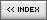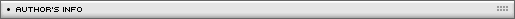Name: Mad Sci Location: N/A Age: N/A Flash experience: N/A Job: N/A Website: http://mad-sci.lazoom.com/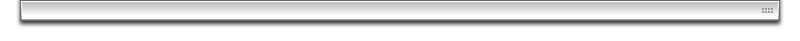| Homepage | News | Games | Articles | Multiplayer Central | Reviews | Spotlight | Forums | Info | Links | Contact us | Advertise | Credits || www.smartfoxserver.com | www.gotoandplay.biz | www.openspace-engine.com | gotoAndPlay() v 3.0.0 -- (c)2003-2008 gotoAndPlay() Team -- P.IVA 03121770048